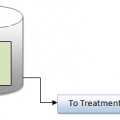## Water Flow Calculation in Pipes

The rate of flow equals the cross-sectional Area of the pipe
Multiplied by the velocity of the water movement Through it, or
Q = AV
Where
Q = volume of flow past a given point per unit of time
A = cross-sectional area of the pipe or conduit
V = velocity of fluid travel in terms of distance per unit of time
Most water distribution systems are sized for a velocity of 3 to 6 ft/s
(0.9 to 1.8 m/s) or for a pressure loss of 1 to 5 lb/in2 per 100 ft of piping.
In this range, flow is turbulent and fine particulates usually stay in suspension.
There is variation in the velocity: pressure loss relationship between small and
large diameter piping,Increased roughness of piping with age, as corrosion or scale
develops, also affects this ratio.
Pipe fittings and valves introduce additional pressure loss, which
provides a basis for estimating overall pressure loss and pumping requirements
for water distribution systems.
Wastewater collection piping is usually larger than freshwater distribution
systems because it frequently must carry off its flow by gravity alone.## Author:T.P.Sivanandan

#### Why Fine Bubbles are Better than Coarse bubble in Aeration Tank#### How to Calculate Lime Dosage Requirement in Sedimentation Process